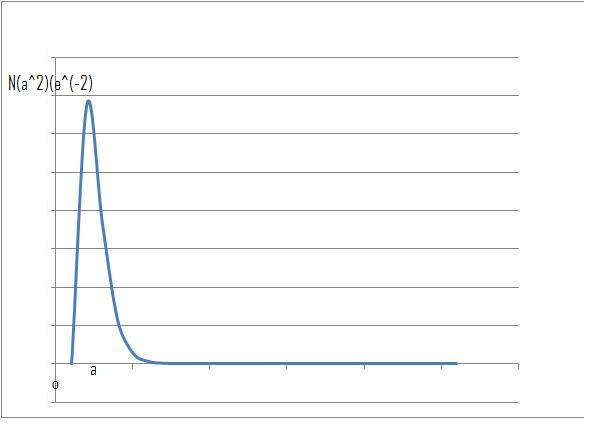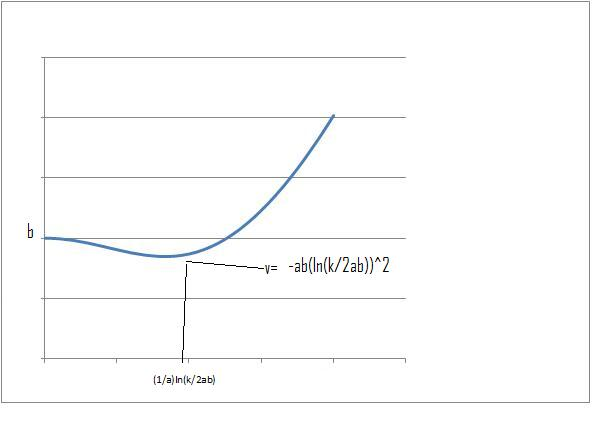#### You may also like### 2D-3D

Two circles of equal size intersect and the centre of each circle is on the circumference of the other. What is the area of the intersection? Now imagine that the diagram represents two spheres of equal volume with the centre of each sphere on the surface of the other. What is the volume of intersection?### Power Up

Show without recourse to any calculating aid that 7^{1/2} + 7^{1/3} + 7^{1/4} < 7 and 4^{1/2} + 4^{1/3} + 4^{1/4} > 4 . Sketch the graph of f(x) = x^{1/2} + x^{1/3} + x^{1/4} -x### Fractional Calculus I

You can differentiate and integrate n times but what if n is not a whole number? This generalisation of calculus was introduced and discussed on askNRICH by some school students.

# Scientific Curves

##### Age 16 to 18Challenge Level

1. A radial probability density function for an electron orbit

$$f(r) = Nr^2e^{-\frac{2r}{a}}$$

I feel that the easiest way to think of this curve is to first consider the curves $r^2$ and $e^-r$ separately, then consider the shape of the curve produced when multiplying the two together.

$r^2$ grows with r whilst $e^{-r}$decays to 0 with increasing r

when $r$ is very small $e^{-r}$ $\approx$ 1, therefore the curve is effectively $r^2$.
when $r \to \infty$ then $e^{-r} \to 0$, when we multiply the two numbers together a very small number will therefore result.

At $r = 0$, $f(r) = f(0) = 0$

at $f(r) = 0$
either $r^2= 0$ therefore $r = 0$
or $e^{\frac{-2r}{a}} = 0$ therefore $r =\ln(0) = \infty$
as $r \to \infty$ we have $f(r) \to 0$

If we now differentiate the function we will be able to find its turning points

$$\frac{d}{dr} Nr^2e^{-\frac{2r}{a}}= 2Nr(1 - \frac{r}{a})e^{\frac{-2r}{a}}$$

When $\frac{d}{dr} = 0$ we find $r =0$, $r= a$ and $r = \infty$.

If we now differentiate once more to determine the nature of each of the turning points we find that:

$$\frac{d^2f}{dr^2}= \frac{d}{dr}2Nr(1 - \frac{r}{a})e^{\frac{-2r}{a}} =2(1-\frac{r}{a} + )(2r)(\frac{-1}{a}))Ne^{\frac{-2r}{a}} + (\frac{-2}{a}(2r)(1 - \frac{r}{a})Ne^{\frac{-2r}{a}}$$

at $r = 0$: $\frac{d^2f}{dr^2}=2N$ which is greater than $0$, therefore $r = 0$ is a minimum

at $r = \infty$: $\frac{d^2f}{dr^2}= 0$

at $r = a$: $\frac{d^2f}{dr^2}=-2Ne^{-2}$ which is less than $0$, therefore $r = a$ is a maximum.

From the above information we can deduce the general shape of $f(r)$ as shown below.
(Note: The graph below has been plotted assuming all constants equal 1 )2. Potential energy for the vibrational modes of ammonium

$$V(x)=\frac{1}{2}kx^2+be^{-ax^2}$$

When $x$ is very small $v(x) \approx be^{-ax^2}$
and when $x$ is large $e^{-ac^2} \to 0$, $v(x) \approx \frac{1}{2}kx^2$

when $x = 0$, $v(0) = b$ by inspection it can be deduced that $v(x)$ can never reach $0$ as $be^{-ax^2}$ is always greater than $0$ and $\frac{1}{2}kx^2$ is greater than $0$ at all $x$ except $x=0$, but at this point $be^{-ax^2}= b$

as $x \to \infty$ we have $v(x) \to \frac{1}{2}kx^2 \to \infty$

If we now differentiate the function we will be able to determine its turning points.

$$\frac{dv}{dx} = xk - 2abxe^{-ax^2} = x\left(k-2abe^{-ax^2}\right)$$

when $\frac{dv}{dx} = 0$ we find $x = 0$ and $(k-2abe^{-ax^2}) =0$
therefore $x = \sqrt{\frac{-1}{a}\ln\left(\frac{k}{2ab}\right)}$

If we now differentiate once more to determine the nature of each of the turning points we find that:

$$\frac{d^2v}{dx^2}= k - 2abe^{-ax^2} + 4a^2bx^2e^{-ax^2}$$

at $x = 0$, $\frac{d^2v}{dx^2}= k- 2ab$ , the nature of this turning point will therefore depend on the relative magnitudes of the constants $a$, $b$ and $k$. If $k$ is greater than $2ab$, $x=0$ will be a minimum but if $2ab$ is greater than $k$ it will be a maximum.

at $x= \sqrt{\frac{-1}{a}\ln\left(\frac{k}{2ab}\right)}$ we should expect a minimum (or possibly a point of inflexion) as a consequence of the fact that as there are only two turning points and as $x \to \infty$ $v(x) \to \infty$ (it therefore cannot be a maximum).